# 有关动态规划【以下内容仅为本人在学习中的所感所想，本人水平有限目前尚处学习阶段，如有错误及不妥之处还请各位大佬指正，请谅解，谢谢！】

#Updated【2022.4.27 修正所附(01背包)代码的错误】

1.    重叠子问题：每一步，在操作上都具有明显的相同相似性。

2.    最优子结构：最终问题的最优解基于每一步的最优解而得出。

• 大化小；将完整的问题划分到每一步
• 分情况定变量，将每一步可做的选择列出；一般用数组值代表最终问题的答案，数组每个索引表示影响到最终答案的变量，类似于自变量与因变量。
• 推方程；在每一个选择的基础上推出当前一步与上一步(或前几步)间的关系
• 定边界；预处理边界，给定初始值

（1）01背包

（2）买卖股票

来源：力扣（LeetCode）

（3）最长回文子串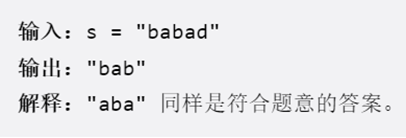来源：力扣（LeetCode）

（4）编辑距离

来源：力扣（LeetCode）

（1）01背包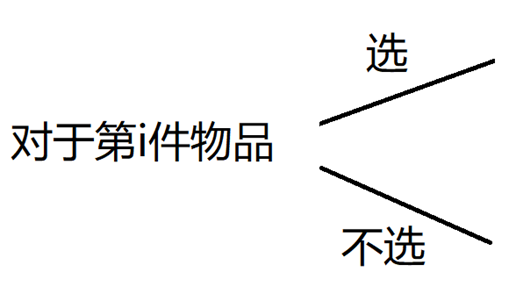（2）买卖股票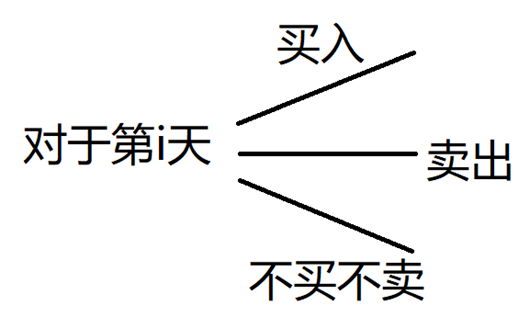（3）最长回文子串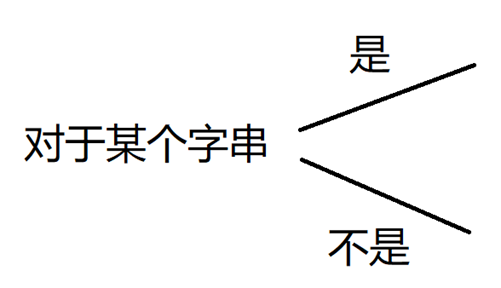（4）编辑距离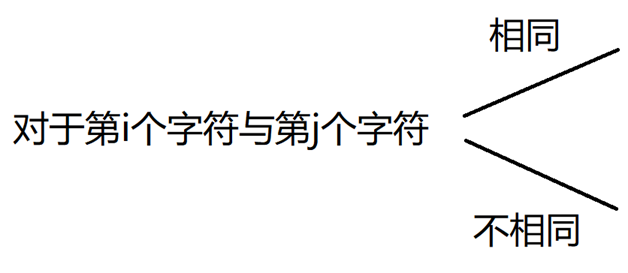（1）01背包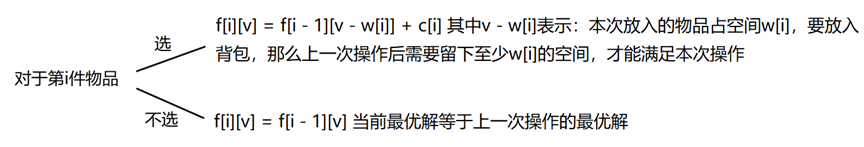（2）买卖股票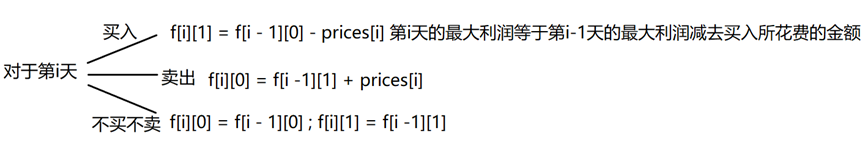（3）最长回文子串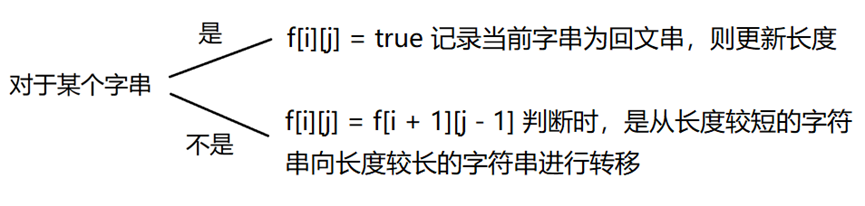（4）编辑距离（1）01背包

(2)  买卖股票

(3)  最长回文子串

（4）编辑长度

【感谢您可以抽出时间阅读到这里，第一篇博客可能会有许多不妥之处；受限于水平，许多地方可能存在错误，还请各位大佬留言指正，请见谅，谢谢！】

#附文中所提到的4个题目的代码

（1）01背包

```#include <bits/stdc++.h>
using namespace std;
int n,v,c,w,f;

int main() {
cin>>n>>v;
for(int i = 1 ;i<=n ;i++)
cin>>w[i];
for(int i = 1 ;i<=n ;i++)
cin>>c[i];
for(int i = 1 ;i<=n; i++) {
for(int j = 1; j<=v ;j++) {
f[i][j] = f[i-1][j];
if(j-w[i]>=0)
f[i][j] = max(f[i-1][j-w[i]]+c[i],f[i][j]);
}
}
cout<<f[n][v]<<endl;
return 0;
}```

（2）买卖股票

```public class Solution {
public int MaxProfit(int[] prices) {
int[,] profit = new int[prices.Length, 2];
profit[0, 1] = -prices;
profit[0, 0] = 0;
for(int i = 1; i < prices.Length; i++) {
profit[i, 1] = Math.Max(profit[i - 1, 1], profit[i - 1, 0] - prices[i]);
profit[i, 0] = Math.Max(profit[i - 1, 0], profit[i - 1, 1] + prices[i]);
}
return profit[prices.Length - 1, 0];
}
}```

（3）最长回文子串

```public class Solution {
public string LongestPalindrome(string s) {
int len = s.Length, begin = 0, maxLen = 1;
if(len < 2) return s;
bool[,] dp = new bool[len, len];//[i, j]是否为回文串
for(int i = 0; i < len; i++) dp[i, i] = true;
for(int L = 2; L <= len; L++)
{
for(int i = 0; i < len; i++)//L = j - i + 1;
{
int j = L + i - 1;
if(j >= len) break;
if(s[i] != s[j]) dp[i, j] = false;
else
{
if(j - i < 3) dp[i, j] = true;
else dp[i, j] = dp[i + 1, j - 1];
}
if(dp[i, j] && j - i + 1 > maxLen)
{
maxLen = j - i + 1;
begin = i;
}
}
}
return s.Substring(begin, maxLen);
}
}```

（4）编辑距离

```public class Solution {
public int MinDistance(string word1, string word2) {
int n = word1.Length, m = word2.Length;
int[,] cost = new int[n + 1, m + 1];
for(int i = 0; i <= n; i++) cost[i, 0] = i;
for(int j = 0; j <= m; j++) cost[0, j] = j;
for(int i = 1; i <= n; i++)
for(int j = 1; j <= m; j++) {
if(word1[i - 1] == word2[j - 1]) cost[i, j] = cost[i - 1, j - 1];
else cost[i, j] = Math.Min(cost[i - 1, j - 1], Math.Min(cost[i, j - 1], cost[i - 1, j])) + 1;
}
return cost[n, m];
}
}```

TRANSLATE with
x

TRANSLATE with
COPY THE URL BELOW

EMBED THE SNIPPET BELOW IN YOUR SITE

<input id="EmbedSnippetTextBox" type="text" name="EmbedSnippetTextBox" value="